NCERT Class 7 Maths Visualizing Solid Shapes

# NCERT Class 7 Maths Visualizing Solid Shapes

The chapter 15 begins with an introduction to plane figures and solid shapes.The next topic of discussion is Faces, Edges and Vertices which is described in detail.Nets for building 3-D shapes is explained in the next section.Drawing solids on a flat surface such as Oblique Sketches, Isometric Sketches, and visualising solid objects are explained in the subsequent sections.Viewing different sections of a solid through various techniques such as  slicing game, kitchen play ,shadow play  and by looking at it from certain angles to get different views is the last topic explained in the chapter.

Download FREE PDF of Chapter-15 Visualizing Solid Shapes

## Chapter 15 Ex.15.1 Question 1

Identify the nets which can be used to make cubes (cut out copies of the nets and try it):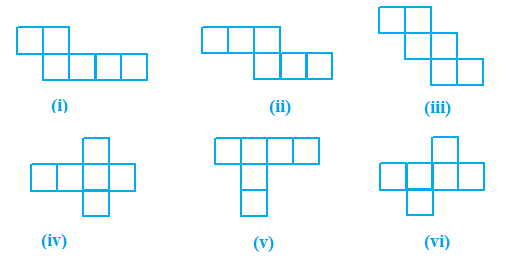### Solution

What is known?

Different nets.

What is unknown?

Which nets can be used to make cube.

Reasoning:

This question is based on the concept of $$3D$$ shapes

Steps:

Figure (ii), (iii), (iv) and (vi) will make cubes. As we know a cube has $$6$$ faces, $$12$$ edges and $$8$$ vertices. So, when we will fold the nets of shapes in (ii), (iii), (iv) and (vii) we will be able to make a cube.

## Chapter 15 Ex.15.1 Question 2

Dice are cubes with dots on each face. Opposite faces of a die always have a total of seven dots on them.

Here are two nets to make dice (cubes); the numbers inserted in each square indicate the number of dots in that box.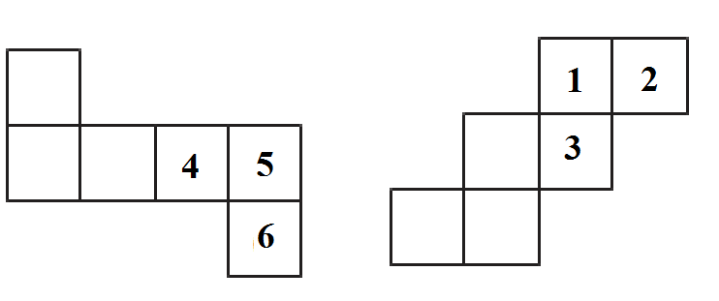Insert suitable numbers in the blanks, remembering that the number on the opposite faces should total to $$7$$.

### Solution

What is known?

Two nets of a dice on which number of dots on some faces are given.

What is unknown?

Number in the squares where there are blanks.

Reasoning:

As we know dice are cubes with dots on each face. Opposite faces of a die always have a total of seven dots on them. Also, in this question nets to make dice (cubes) is given along the numbers inserted in each square indicate the number of dots in that box.

Steps:

In figure (i) $$1^{\rm st}$$ blank net will be number $$1$$ in dice because opposite net to it will be $$6$$ as given. $$2^{\rm{nd}}$$ net will be $$3$$ as opposite to it will be $$4$$ as given and last $$3^\rm{rd}$$ net will be $$2$$ as opposite to it will be $$5$$.

In figure (ii) $$1^ \rm {st}$$ blank net will be no. $$5$$ in dice because opposite net to it will be $$2$$ as given. $$2^\rm{nd}$$ net will be $$6$$ as opposite to it will be $$4$$ as given and last $$3^\rm{rd}$$ net will be $$4$$ as opposite to it will be $$3$$.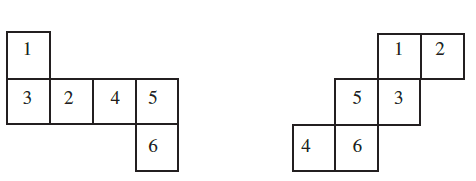## Chapter 15 Ex.15.1 Question 3

Can this be a net for a die?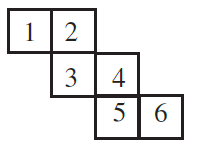### Solution

What is known?

A net.

What is unknown?

Can this be a net for a dice.

Reasoning:

Dice is a cube with dots on each face. Opposite faces of a die always have a total of seven dots on them. Make a cube of this net and if opposite faces of this cube have sum of $$7$$ then it can be a net for dice.

Steps:

No, because we know that dice is a cube with dots on each face. Opposite faces of a die always have a total of seven dots on them.

So, if we will fold this net opposite to $$4$$ will be $$1$$ and opposite to $$3$$ will be $$6$$ which does not make total of $$7.$$

## Chapter 15 Ex.15.1 Question 4

Here is an incomplete net for making a cube. Complete it in at least two different ways. Remember that a cube has six faces. How many are there in the net here? (Give two separate diagrams. If you like, you may use a squared sheet for easy manipulation.)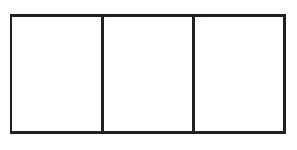### Solution

What is known?

Incomplete net for a cube.

What is unknown?

Complete net for a cube in two different ways.

Reasoning:

Three faces of cube have given so we can implement other three faces in this way to get a completed cube.

Steps: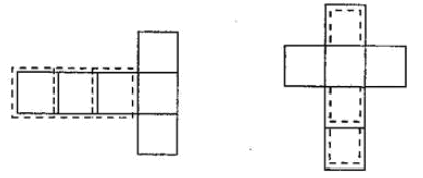## Chapter 15 Ex.15.1 Question 5

Match the nets with appropriate solids: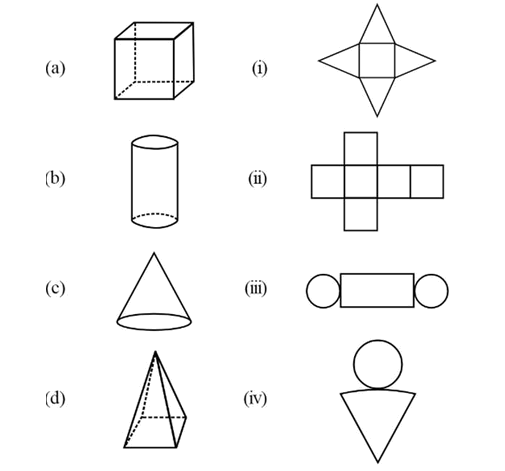### Solution

What is known?

Nets and solids

What is unknown?

Matching the nets with appropriate solids.

Reasoning:

Match the solids to their appropriate nets.

Steps: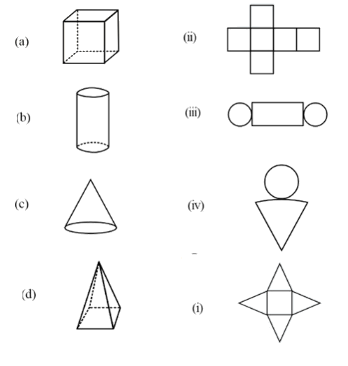Visualising Solid Shapes | NCERT Solutions
Instant doubt clearing with Cuemath Advanced Math Program